## Sum values based on column or both column and row

This tutorial provides different formulas based on the SUMPRODUCT function to help you sum values based on a column header, and sum values based on both column and row criteria.

How to sum values based on column header?
How to sum values based on both column and row criteria?

#### How to sum values based on column header?

Let’s look at the following example, there is a products’ monthly sales table in range B4:H10, to sum only the sales in the month “Mar”, you can do as follows.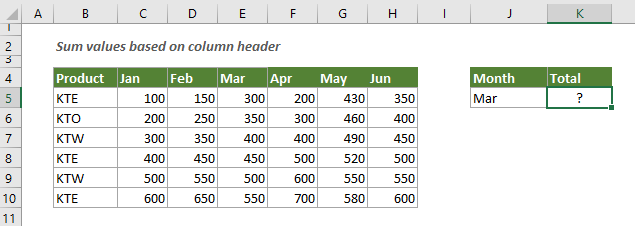Generic Formulas

Arguments

Columns: The range of columns (excluding the headers) that you want to sum only the specific one. In this example, it is the range C5:H10;

How to use this formula?

Select a blank cell, copy or enter the formula below into it and press the Enter key to get the result.

=SUMPRODUCT((C5:H10)*(C4:H4=J5))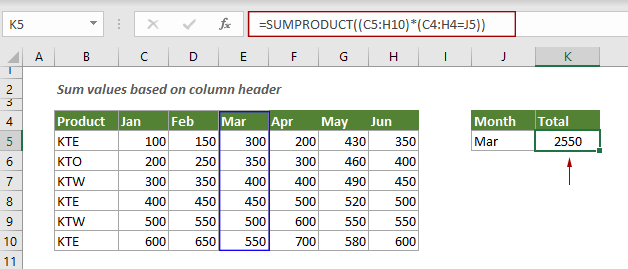Note: In the formula, J5 is the cell reference to the specific column heading. You can type the column heading directly into the formula and enclose it in double-quotes.

=SUMPRODUCT((C5:H10)*(C4:H4="Mar"))

Explanation of the formula

=SUMPRODUCT((C5:H10)*(C4:H4=J5))

The SUMPRODUCT function matches all instances of the column heading “Mar” and sums them.

#### How to sum values based on both column and row criteria?

This section will guide you how to sum values based on both the column and row headings in Excel.

As shown in the screenshot below, to sum all sales for “KTE” in the month “Mar”, you can do as follows.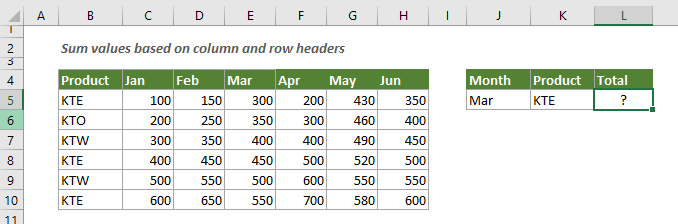Generic formula

Arguments

Columns: The range of columns (excluding the headers) that you want to sum only the specific one. In this example, it is the range C5:H10;

How to use this formula?

Select a blank cell, copy or enter the formula below into it and press the Enter key to get the result.

=SUMPRODUCT((C5:H10)*(C4:H4=J5)*(B5:B10=K5))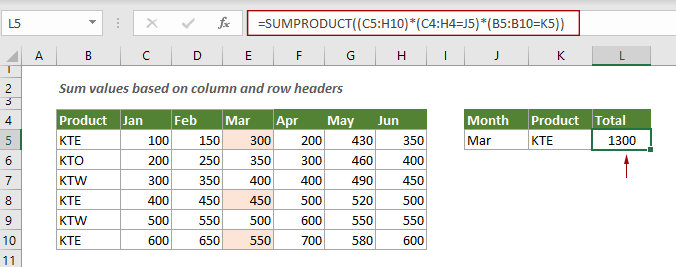Explanation of this formula

=SUMPRODUCT((C5:H10)*(C4:H4=J5)*(B5:B10=K5))

C4:H4=J5: Here checks if each cell in C4:H4 equal to Mar, and returns an array of TRUE and FALSE like this {FALSE;FALSE;TRUE;FALSE;FALSE;FALSE};
(C5:H10)*({FALSE;FALSE;TRUE;FALSE;FALSE;FALSE}): The TURE and FALSE are converted to 1 and 0. Each values in C5:H10 is multiplied by values in the array, after multiplying, you will get another array, here I show the array in the table below: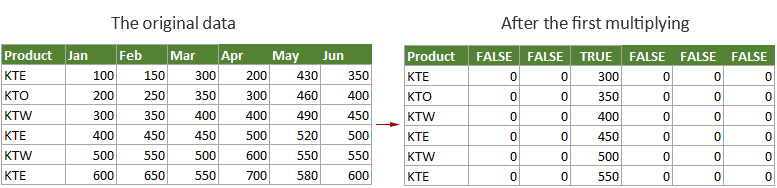B5:B10=K5: Here checks if each cell in B5:B10 equal to KTE, and returns an array of TRUE and FALSE like this {TRUE;FALSE;FALSE;TRUE;FALSE;TRUE};
Then these two arrays multiply together to get a new array as the below table shown.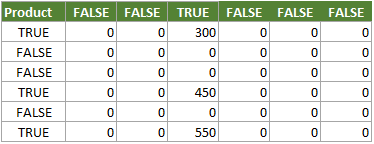Finally, the SUMPRODUCT function sums all values (300+450+550) in the array and returns the final result as 1300.

#### Related functions

Excel SUMPRODUCT function
The Excel SUMPRODUCT function can be used to multiply two or more columns or arrays together, and then get the sum of products.

#### Related formulas

Sum multiple columns if one criterion is met
This tutorial will guide you how to sum values in multiple columns based on the same criterion in Excel.

Sum the last N columns
To sum values in the last n columns, normally you may need to calculate the sum of each column and then add up the results to get the final sum. This tutorial provides a formula based on the INDEX function to help you easily get it done.

Sum top N values or top N values with criteria
This step-by-step tutorial demonstrates how to use the SUMPRODUCT function with the LARGE function to sum the top N values in a range and sum the top N values in a range with criteria in Excel.

Sum values in last N days based on criteria
To sum values in last N days based on specific criteria in Excel, you can apply a formula based on the SUMIF and TODAY function.

### The Best Office Productivity Tools

#### Kutools for Excel - Helps You To Stand Out From Crowd

 Popular Features: Find/Highlight/Identify Duplicates  |  Delete Blank Rows  |  Combine Columns or Cells without Losing Data  |  Round without Formula ... Super VLookup: Multiple Criteria  |  Multiple Value   |  Across Multi-Sheets  |  Fuzzy Lookup... Adv. Drop-down List: Easy Drop Down List  |  Dependent Drop Down List  |  Multi-select Drop Down List... Column Manager: Add a Specific Number of Columns  |  Move Columns  |  Unhide Columns  |  Compare Columns to Select Same & Different Cells ... Featured Features: Grid Focus  |  Design View  |  Big Formula Bar  |  Workbook & Sheet Manager  |  Resource Library (Auto Text)  |  Date Picker  |  Combine Worksheets  |  Encrypt/Decrypt Cells  |  Send Emails by List  |  Super Filter  |  Special Filter (filter bold/italic/strikethrough...) ... Top 15 Toolsets:  12 Text Tools (Add Text, Remove Characters ...)  |  50+ Chart Types (Gantt Chart ...)  |  40+ Practical Formulas (Calculate age based on birthday ...)  |  19 Insertion Tools (Insert QR Code, Insert Picture from Path ...)  |  12 Conversion Tools (Numbers to Words, Currency Conversion ...)  |  7 Merge & Split Tools (Advanced Combine Rows, Split Cells ...)  |  ... and more

Kutools for Excel Boasts Over 300 Features, Ensuring That What You Need is Just A Click Away...

Supports Office/Excel 2007-2021 and 365   |   Available in 44 Languages   |   Easy to Uninstall Completely#### Office Tab - Enable Tabbed Reading and Editing in Microsoft Office (include Excel)

• One second to switch between dozens of open documents!
• Reduce hundreds of mouse clicks for you every day, say goodbye to mouse hand.
• Increases your productivity by 50% when viewing and editing multiple documents.
• Brings Efficient Tabs to Office (include Excel), Just Like Chrome, Edge and Firefox.